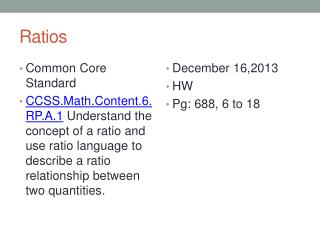DownloadDownload PresentationRatios

# Ratios

Télécharger la présentation## Ratios

- - - - - - - - - - - - - - - - - - - - - - - - - - - E N D - - - - - - - - - - - - - - - - - - - - - - - - - - -
##### Presentation Transcript

1. Ratios • Common Core Standard • CCSS.Math.Content.6.RP.A.1 Understand the concept of a ratio and use ratio language to describe a ratio relationship between two quantities. • December 16,2013 • HW • Pg: 688, 6 to 18

2. Warm up

3. Comparing Ratios and Fraction • Fractions are two term ratios. • Example:

4. Example 1 • The coed soccer team has four time as many boys as it has girls. We say the ratio of the number of the boys to the girls in the team in 4:1. We read this as four to one. • What are some other options that show four times as many as boys as girls or a ratio of boys to girls of 4 to 1? • What are some other option to show that there are three boys for every two girls on the team?

5. Activity • Find the ratio of boys to girls in our class? • Imp Notes: • Is the number of girls to number of boys the same as the ratio of number of boys to girls? (Order matters) • Ratio itself does not have units or word attached

6. Let us record ratios • Your favorite subject is Math • Your favorite subject is LA

7. Using words describe a situation • 1 to 12 • 12 :1 • 2 to 5 • 5 :2 • 10 to 2 • 2:10

8. Problem Solving • To make plaster, Kevin mixes 3 cups of water to make 4 pounds of plaster powder. Make a ratio table to find out how much water will he mix with 20 pounds of water?

9. Independent Practice

10. Closure • A ratio is an ordered pair of non negative numbers which are both non zero. • A ratio is written in A:B to indicate the order of numbers. Switching the numbers changes the relationship • http://learnzillion.com/lessons/602-understand-the-difference-between-fractions-and-ratios